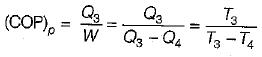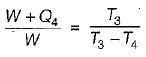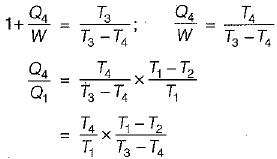Courses

# Test: Second Law of Thermodynamics - 3

## 10 Questions MCQ Test Topicwise Question Bank for Mechanical Engineering | Test: Second Law of Thermodynamics - 3

Description
This mock test of Test: Second Law of Thermodynamics - 3 for Mechanical Engineering helps you for every Mechanical Engineering entrance exam. This contains 10 Multiple Choice Questions for Mechanical Engineering Test: Second Law of Thermodynamics - 3 (mcq) to study with solutions a complete question bank. The solved questions answers in this Test: Second Law of Thermodynamics - 3 quiz give you a good mix of easy questions and tough questions. Mechanical Engineering students definitely take this Test: Second Law of Thermodynamics - 3 exercise for a better result in the exam. You can find other Test: Second Law of Thermodynamics - 3 extra questions, long questions & short questions for Mechanical Engineering on EduRev as well by searching above.
QUESTION: 1

Solution:
QUESTION: 2

### An engine operates between temperature limits of 900 K and T2 and another between T2 and 400 K for both to be equally efficient T2 will be equal to

Solution:

For efficiency to be equal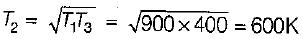QUESTION: 3

### An inventor claims that a heat engine has the following specifications: Developed = 50 kW Fuel burned per hour = 3 kg Heating value of fuel = 7500 kJ/kg Temperature limit = 627°C and 27°C Cost of fuel = Rs. 30/kg Power the performance of his engine is

Solution: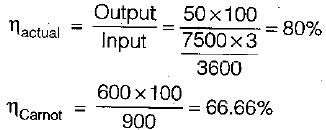Since actual efficiency of the engine is greater than Carnot hence engine is not possible.

QUESTION: 4

Three engines A, B and C operating on Carnot cycle respectively use air, steam and Helium as the working fluid. If all the engine operates within the same temperature limit, then which engine will have highest efficiency.

Solution:

Since efficiency of the engine purely depends upon source and sink temperatures and independent of the working substances. All the engines have same efficiency.

QUESTION: 5

If a heat engine gives an output of 3 kW.when the input is 10000J/sec then the thermal efficiency of the engine will be

Solution: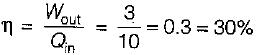QUESTION: 6

A Carnot engine operates between 27°C and 327°C. If the engine produces 300 kJ of work, what is the entropy change during heat rejection?

Solution: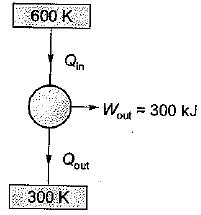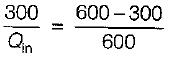Oin = 600 KJ
Qout = 300K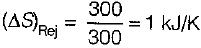QUESTION: 7

The following heat engine produces power of 100,000 kW. The heat engine operates between 800 K and 300 K. It has a thermal efficiency equal to 50% of that of the Carnot engine for the same temperatures. The rate at which heat is absorbed from the hot reservoir is

Solution:

Power produced by engine = Workdone(W) = 100,000 k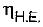= 50% of Carnot cycle or engine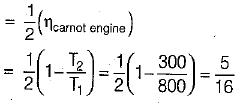Also,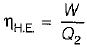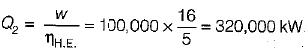= 320 MW

QUESTION: 8

A heat engine operates at 75% of the maximum possible efficiency. The ratio of the heat source temperature (in K) to the heat sink temperature (in K) is 5/3. The fraction of the heat supplied that is converted to work is

Solution:

Given,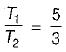Efficiency,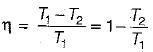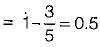Also η = Work done/Heat supplied
Since the engines uses only 75% of the heat made available to it, therefore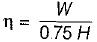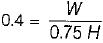W = 0.3 x H

QUESTION: 9

Which of the following represents the Carnot cycle (ideal engine)?

Solution:

Carnot cycle consist of two Isentropics and two isothermals.

QUESTION: 10

A reversible power cycle is used to drive a reversible heat pump cycle. The power cycle takes in Q1 heat units at T1 and reflects Q2 at T2. The heat pump abstracts Q4 from sink at T4 and discharges Q3 at T3. The ratio of Q4/Q1 (in terms of four temperature) is

Solution:

The power cycle operating between temperature T1, and T2 and heat pump is operating between temperature T3 and T4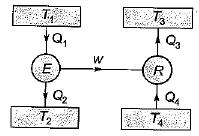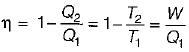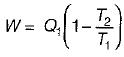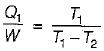COP of reversible pump